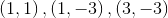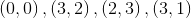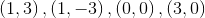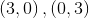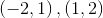# ISEE Lower Level Math : How to find a triangle on a coordinate plane

## Example Questions

### Example Question #17 : Geometry

A coordinate plane is shown.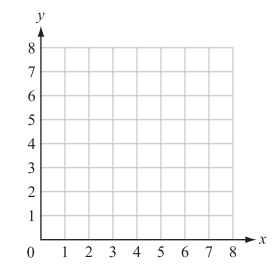Ralph plotted the following points on the coordinate grid:

Point X (7, 0); Point Y (7, 5); Point Z (0, 5)

A polygon is formed with vertices X, Y, and Z. Which type of polygon is formed?

Square

Pentagon

Parallelogram

Triangle

Rectangle

Triangle

Explanation:

Start by graphing and connecting the vertices.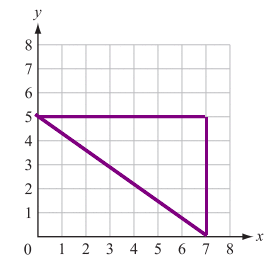The created figure has 3 sides and 3 angles. The only answer choice that has these characteristics is the triangle.

### Example Question #18 : Geometry

A coordinate plane is shown.Ralph plotted the following points on the coordinate grid:

Point X (8, 8); Point Y (1, 4); Point Z (6, 5)

A polygon is formed with vertices X, Y, and Z. Which type of polygon is formed?

Square

Triangle

Rectangle

Triangle

Explanation:

Start by plotting and connecting the ordered pairs.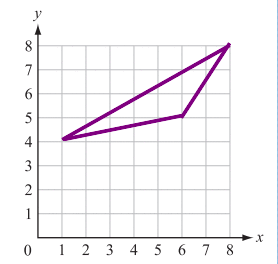The created figure has 3 sides and 3 angles. The only answer choice that has these characteristics is the triangle.

### Example Question #19 : Geometry

Which of the following sets of points would form a triangle if plotted on a coordinate plane?